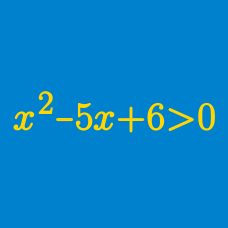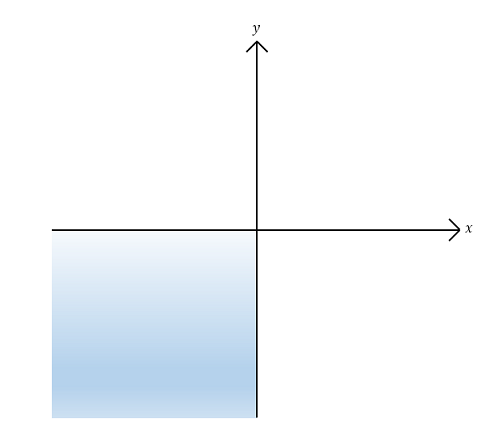Algebra

# Polynomial Inequalities - Sets

$$A$$ and $$B$$ are two subsets of $$\mathbb{R}$$ defined by: \begin{align} A &= \{ x \mid x^2 - 6 x - 91 \leq 0 \}, \\ B &= \{ x \mid x^2 - 6 x - 91 = 0 \}. \end{align} How many integers does the set $$A \cap B^C$$ contain?

Details and assumptions

$$B^C$$ denotes the complement of set $$B$$.

$$\mathbb{R}$$ denotes the set of all real numbers.Sets $$A, B, C$$ and $$D$$ are defined as follows: \begin{align} A &= \{ (x,y) \vert x>0 \} \\ B &= \{ (x,y) \vert y>0 \} \\ C &= \{ (x,y) \vert y>5x \} \\ D &= \{ (x,y) \vert y<-3x \}. \end{align} Then which of the following is the set that represents the shaded region, including the two axes, in the above graph?

The sets $$A = \{ x \mid x^2 - 5 x + 4 \leq 0 \}$$ and $$B = \{ x \mid x^2 + ax + b < 0 \}$$ satisfy the following conditions: $A \cap B = \emptyset \mbox{ and } A \cup B = \{ x \mid 1 \leq x < 15 \}.$ What is the value of $$a + b?$$

$$P,\ Q$$ and $$R$$ are three subsets of $$\mathbb{R}$$ defined as follows: \begin{align} P &= \{ x \mid x^2 - 21x + 110 > 0 \}, \\ Q &= \{ x \mid x^2 - 15x + 44 < 0 \}, \\ R &= \{ x \mid x^2 - 11ax + 10a^2 < 0,\ a > 0 \}. \end{align} What is the sum of the minimum and maximum values of $$a$$ such that $$(P \cap Q) \subset R$$?

Details and assumptions

$$\mathbb{R}$$ denotes the set of all real numbers.

$$A$$, $$B$$ and $$C$$ are subsets of $$\mathbb{R}$$ defined as follows: \begin{align} A &= \{ x \mid x^2 - 10 x + 21 \geq 0 \}, \\ B &= \{ x \mid x^2 - 10 x + 21 > 0 \},\\ C &= \{ x \mid x^2 - 19 x + 48 = 0 \}. \end{align} What is the value of the sum of all the elements of the set $$(A \cap B^C) \cup C$$?

Details and assumptions

$$B^C$$ denotes the complement of set $$B$$.

$$\mathbb{R}$$ denotes the set of all real numbers.

×

Problem Loading...

Note Loading...

Set Loading...